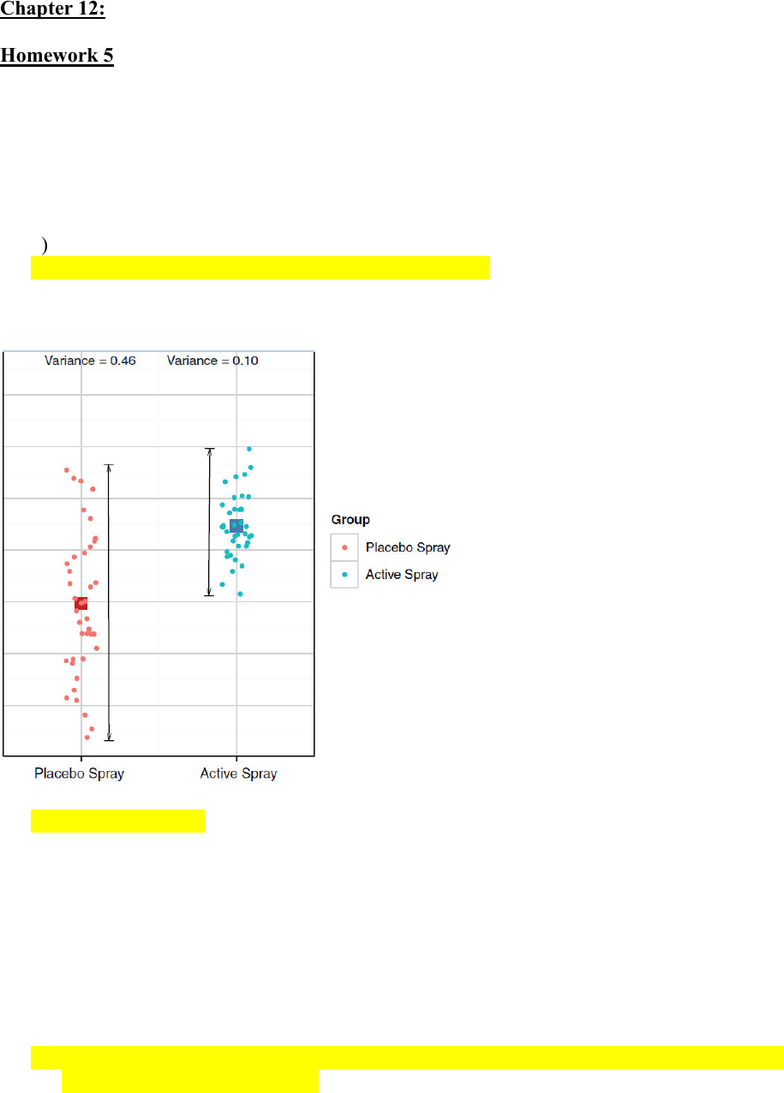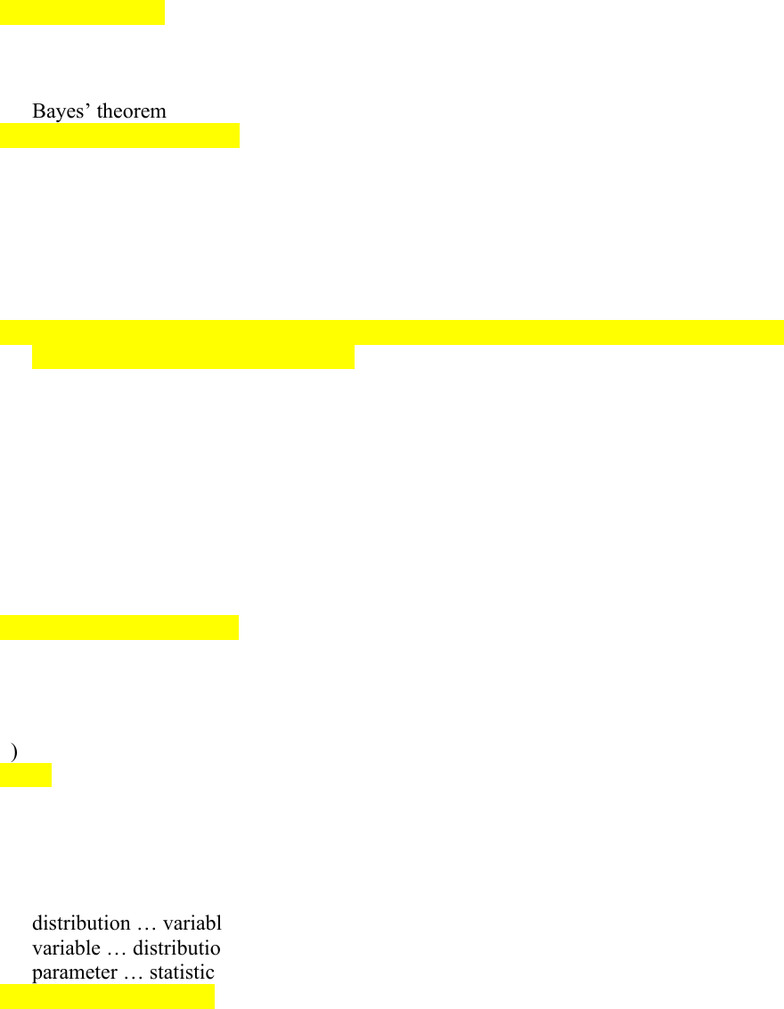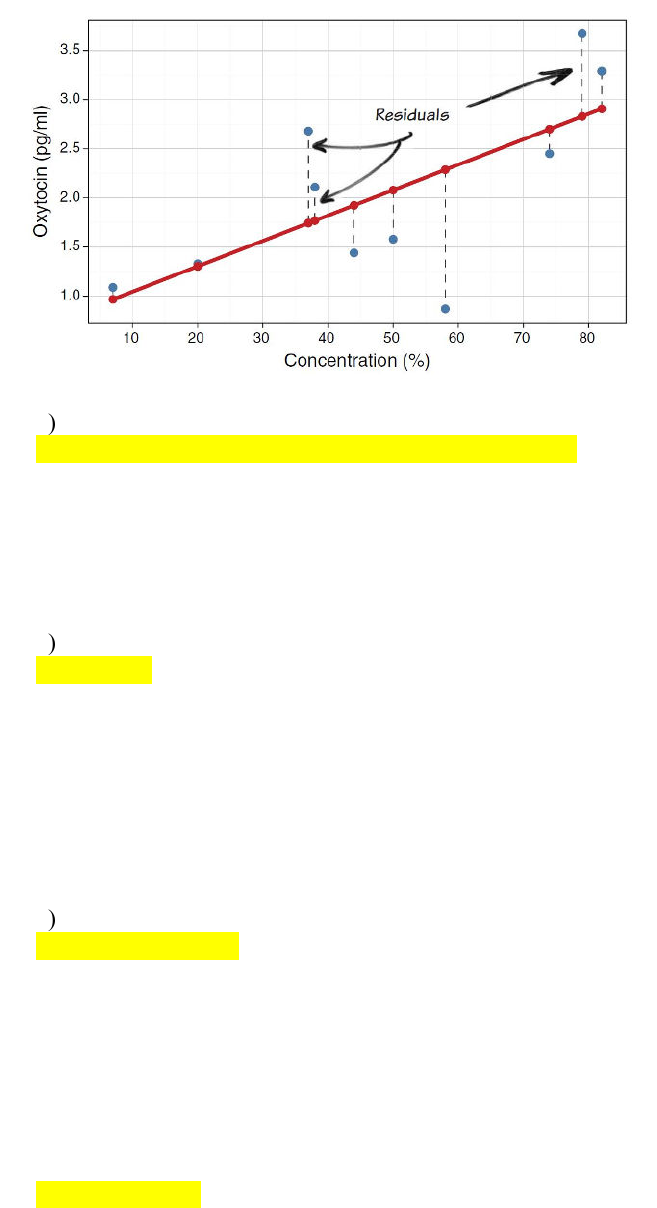# PNB 3XE3 Study Guide - Quiz Guide: Homoscedasticity, General Linear Model, Multicollinearity

52 views11 pages
School
McMaster University
Department
Psychology, Neuroscience & Behaviour
Course
PNB 3XE3Chapter 12:
Homework 5
1. We are interested in whether ___ is a good fit to the observed data.
a) our model, for which parameters have been calculated based on our data
b) b0 + b1Xi, where b1 is a parameter describing the relationship between our independent
variable and the outcome or dependent variable
c) b0 + b1X1i + b2X2i + … bnXni
d) most generally, an equation that summarizes the pattern of observed data
2. The following graph shows a violation of which assumption?
a) homoscedasticity
b) no perfect multicollinearity
d) independent errors
a) you can add one model to another
b) you can add equations together
c) you can add outcome variables together
d) if a number of variables affect the outcome, then their combined effect is best described
Unlock document

This preview shows pages 1-3 of the document.
Unlock all 11 pages and 3 million more documents.4. With the equation outcomei = (b0 + b1Xi) + errori …
a) the parameters are estimated based on your collected data
b) for each parameter, you can calculate a test statistic
c) for each parameter you can calculate a p-value
d) all of the above
5. The equation outcomei = (b0 +b1Xi) + errori is called
a) Bayes’ theorem
b) the general linear model
c) the equation
d) the hypothesis test
6. In which example would errors be independent?
a) You have people watching a movie and then interview them to get their ratings. After
watching the movie together, they line up to wait until you call them one by one.
b) You are measuring reaction time while observers are alone in your lab. Several students
who enroll are in the same stats class.
c) You are measuring Netflix binging as an effect of household income. A married couple
d) You are interested in people’s ratings of the new psychology curriculum. You increase
your sample size by having enrolled participants bring their friends.
7. Which of the following is false?
a) you use a statistical model to predict the outcome for a given case (i)
b) a model is derived from a specific data set
c) a model is always imperfect
d) a model is always linear
8. In the equation outcomei = (b0 + b1Xi) + errori which is the predictor variable?
a) b1Xi
b) outcomei
c) Xi
d) errori
9. A ___ is a numerical characteristic of a sample and a ___ is a numerical
characteristic of a population.
a) distribution variable
b) variable distribution
c) parameter statistic
d) statistic … parameter
Unlock document

This preview shows pages 1-3 of the document.
Unlock all 11 pages and 3 million more documents.10. In the following figure, each blue dot represents what?
a) the error
b) parameters
c) the level of oxytocin measured in a particular person
d) the residual
11. If the relationship between the predictor variable and the outcome variable can be
best described by a straight line, you have satisfied which of the following?
a) homoscedasticity
b) no perfect multicollinearity
c) linearity
d) predictors are uncorrelated with external variables
12. You measure vocabulary in a group of 2 year olds, a group of 10 year olds and a
group of 18 year olds. You find little variance among the 2 year olds, a bit more
among the 10 year olds, and a great deal of variance among the 18 year olds. This
data set violates which assumption?
b) independent errors
c) homoscedasticity
d) all of the above
13. The difference between the value of the outcome predicted by the model and the
value of the outcome actually observed is called the…
a) error
b) residual
c) variance
d) A and B only
Unlock document

This preview shows pages 1-3 of the document.
Unlock all 11 pages and 3 million more documents.

## Document Summary

After watching the movie together, they line up to wait until you call them one by one: you are measuring reaction time while observers are alone in your lab. Several students who enroll are in the same stats class: you are measuring netflix binging as an effect of household income. A married couple enrolls in your study: you are interested in people"s ratings of the new psychology curriculum. You find little variance among the 2 year olds, a bit more among the 10 year olds, and a great deal of variance among the 18 year olds. A researcher wanted to know exactly what it was about being a professor that created this stress and subsequent burnout. She recruited 75 professors and administered several questionnaires that measured: burnout (high score = burnt out), perceived. The outcome of interest was burnout, and cooper"s (1988) model of stress indicates that perceived control and coping style are important predictors of this variable.